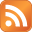# The Simpsons Hit & Run PS2 cheatsCheat codes

Enter the options screen in either the main menu or during play, hold L1 and R1, keeping them held down enter one of the following codes (the sound of a horn will confirm correct code entry):

Drunken Mode - triangle, circle, triangle, circle
Blow up cars on impact - triangle, triangle, square, square
Invincible car - triangle, X, triangle, X
High acceleration - triangle, triangle, triangle
No top speed - square, square, square, square
Night time mode - X, X, X, X
Press L3 to make car jump - square, square, square, triangle
Speedometer - triangle, triangle, circle, square
Turn rocket-car into red-brick-car - circle, circle, triangle, square
All reward cars - X, circle, X, circle
Grid Mode - circle, X, circle, triangle
More camera angles - circle, circle, circle, X
Alternate Credits audio - X, square, square, triangle